# Music Instrument Recognition Using Neural Networks

Working with audio data is both fascinating and challenging at the same time. But what makes it difficult to process audio data? Unlike images, sound data can not be represented in tabular format (Structured data), and our models are not designed to handle unstructured data. To make this heavy task look simple, we will discuss some approaches to convert the audio data into a feedable form for the Artificial Neural Network in this blog.

### Key Takeaways

1. Methods of Audio Classification: Spectrograms and MFCCs
2. RGB v/s Grayscaled images.
3. Steps for Audio classification using ANN model.
4. Applications of audio classification.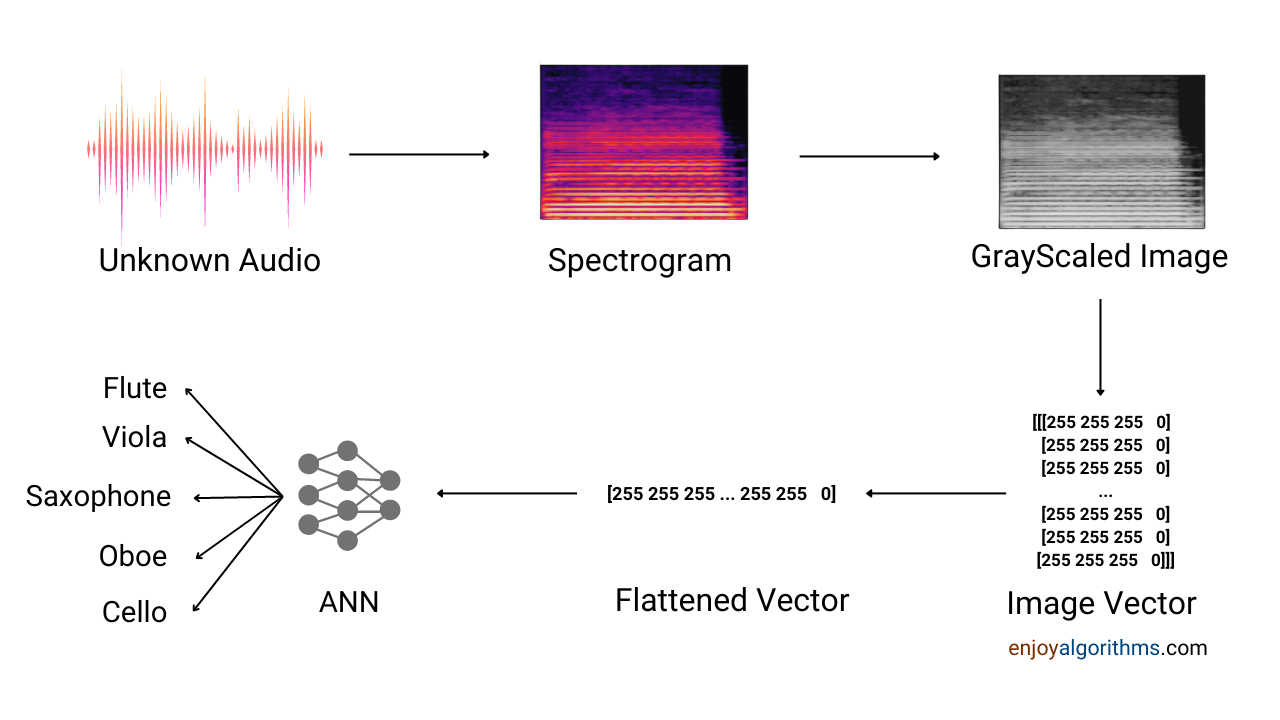### Methods of Audio Classification

Music Information Retrieval(MRI) is the standard term used for audio classification. It is the extraction of information from the audio data and converting it to machine-feedable form without any loss of information bits. We are still unable to make models that can take audio files as input and process them.

Apart from this, audio data also needs more space and is difficult to process. Therefore researchers and data professionals keep exploring and implementing new methods to deal with audio data. The most famous among these techniques are Spectrogram and MFCCs of audio.

#### Spectrograms

A spectrogram is a frequency spectrum of an audio signal varying with time. It is a 2D graph representing the frequency, amplitude, and time. Frequency is shown on the vertical axis, time is on the horizontal axis, and the variation of colours represents amplitude. Dark red or orange for higher amplitudes and dark blue for small amplitudes. The variation of these three parameters is unique for each signal.

The mathematical way of calculating spectrograms is using Short Time Fourier Transform (STFT). Fourier Transform converts the signal from its time domain to the frequency domain making the signal analysis easier. To calculate Fourier transform efficiently, we use Fast Fourier Transform.

But this approach also fails when it comes to time-varying signals like speech or instrument music. The signal, in this case, changes continuously thus, applying FFT over the whole signal once will not give the correct results. Here comes the Short-Time Fourier Transform (STFT). It calculates the Fourier transform over various windows and merges them to represent the Frequency spectrum of the time-varying signal.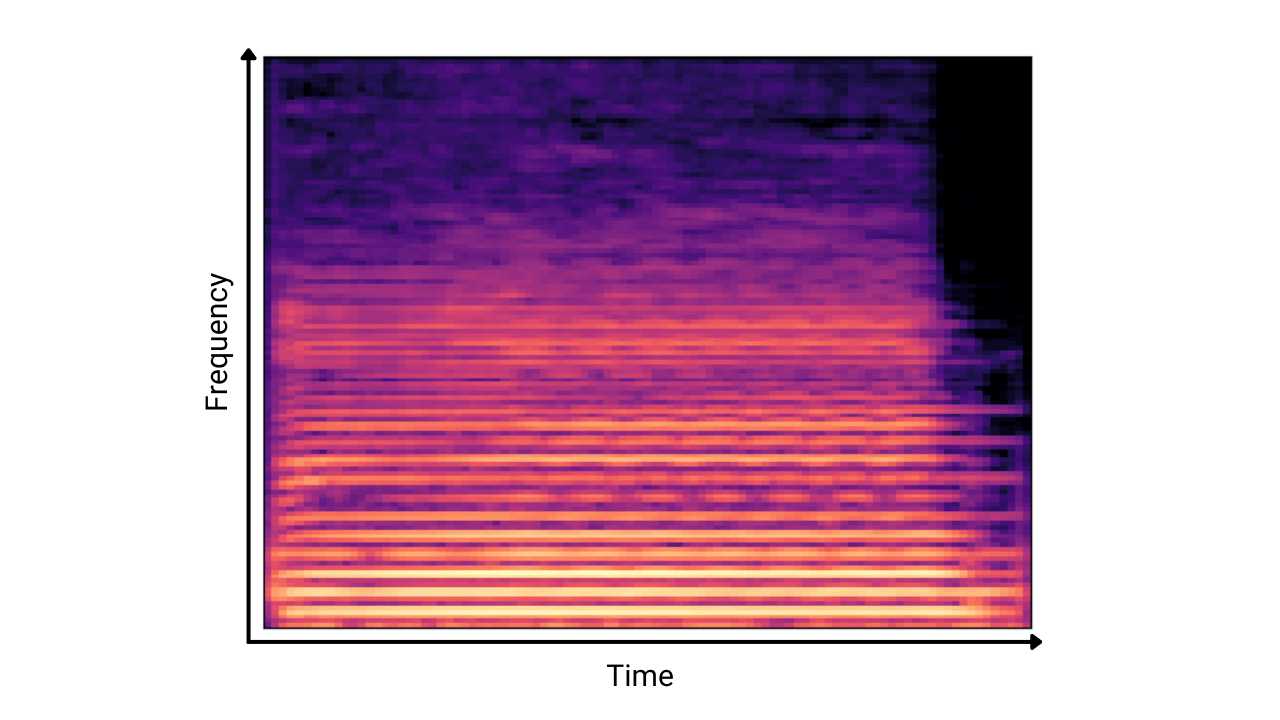The idea was to find the spectrogram of the audio data and then convert that to an image vector to feed it to the neural networks. In this way, we can reduce the space used to store the audio data and make a model using the same technique we use for tabular data.

#### Mel-frequency cepstral coefficients (MFCCs)

Mel-frequency cepstral is a close-packed power spectrum of an audio signal. It is calculated by dividing the signal into small frames and then windowing it to apply the Short-Time Fourier Transform.

After applying STFT and converting the frequency according to the mel-scale(logarithmic scale for frequency), the signal is passed through a combination of filters, generally called a filter bank containing 40 filters in general. It provides a vector containing the negative and positive coefficients.

The positive value of the coefficient represents the low-frequency region, and the negative value means the high-frequency region. This vector representation is unique for each audio and can be directly used to train the model.

Both these techniques of audio classification are trusted and tested, and we will discuss and compare both in this blog.

### How to make Spectrograms?

First, we must import the libraries that read and process audio data. Librosa is one such library. It allows us to read, display and analyze audio files. We will use Librosa’s Mel spectrogram() function to convert audio to spectrograms. The dataset can be found at this link.

``````def mel_spectrogram(audio_files):

image_path = fullPath + 'spectrograms/'
try:
os.makedirs(image_path)
except FileExistsError as exception:
print('')

for audio in audio_files:
print("done")
y = audio
sr = audio
file = audio
# How to make Spectrogram
S = librosa.feature.melspectrogram(y=y, sr=sr)
fig= plt.plot()
S_dB = librosa.power_to_db(S, ref=np.max)
img = librosa.display.specshow(S_dB, sr=sr)
plt.savefig(image_path + file[:-4] + '.png', transparent=True)``````

We started with storing the audio files with their names and specifications in an array audiofiles. We then fed this array to the melspectrogram function. Let’s see the parameters involved in this function.

librosa.feature.melspectrogram(*\,* y=None, sr=22050, S=None, nfft=2048, hoplength=512, winlength=None, window=’hann’, center=True, padmode=’constant’, power=2.0, **kwargs*)**

We need two pieces of information for creating spectrograms: the amplitude variation with time and the signal’s sampling rate. Y is the time series data of the audio, and sr is the sampling rate of the signal. If Y and sr are provided, then the function plots the spectrogram, and if the spectrogram(S) is given, then it just maps it onto the mel scale.

#### What is Mel Scale?

Mel scale is the logarithmic scale for frequency. This logarithmic transformation is done to replicate human nature. For us, it is easy to tell that 100HZ frequency sounds nearer than 200Hz, but we might fail to differentiate between 10000Hz and 10100Hz. It happens because we perceive the logarithmic scale to distinguish between the pitches. Therefore we are using Mel Spectrograms to blend human nature into our Neural Network model.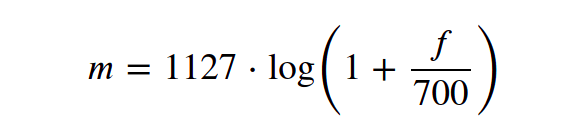The formula for mel-scale conversion

Understanding mel-scale was important, right? Now let’s see what else we need to make spectrograms.

Next, we need to create the axis handle to plot the spectrogram. Librosa.display.specshow() gives us the axis handle. It takes the frequency spectrum returned by the melspectrogram function in the form of an array and transforms it into a graph, which we call the spectrograms. After plotting the graph, we saved it in the corresponding folder.

This was one method to approach any audio classification problem. Now, let’s see the mel-frequency cepstral method.

### How to find MFCCs?

There is only a slight difference between finding the spectrograms and MFCCs. We need the same time series audio data and sample rate of the audio signal and have to define the number of coefficients (generally 40) to calculate the MFCCs.

MFCCs are preferable when we have storage and time constraints. It delivers the same results as spectrograms, and the coefficients are easy to find.

``````extracted_features=[]
for file in files:

if '.mp3' in file:
name=file.split('/')[-1].split('_')
audio_files = []
mfccs_features = librosa.feature.mfcc(y=audio, sr=sample_rate, n_mfcc=40)
mfccs_scaled_features = np.mean(mfccs_features.T,axis=0)
audio_files.append(mfccs_scaled_features)
extracted_features.append([mfccs_scaled_features,name])

extracted_features_df=pd.DataFrame(extracted_features,columns=['feature','class'])
X=np.array(extracted_features_df['feature'].tolist())
y=np.array(extracted_features_df['class'].tolist())``````

We first apply the Fourier transform to get the signal's frequency spectrum. Then we convert it to the power spectrum by taking the square of the values at each frequency. Now to reflect the human nature of hearing, we need to scale the power spectrum onto the logarithmic scale commonly known as the mel-scale. It is done by passing the spectrum through a mel-scaled filter bank consisting of 20–40 overlapping triangular band-pass filters.

After plotting the power spectrum over the mel-scale, we take the Discrete Cosine Transform or Inverse Discrete Fourier Transform to get the mel-frequency coefficients.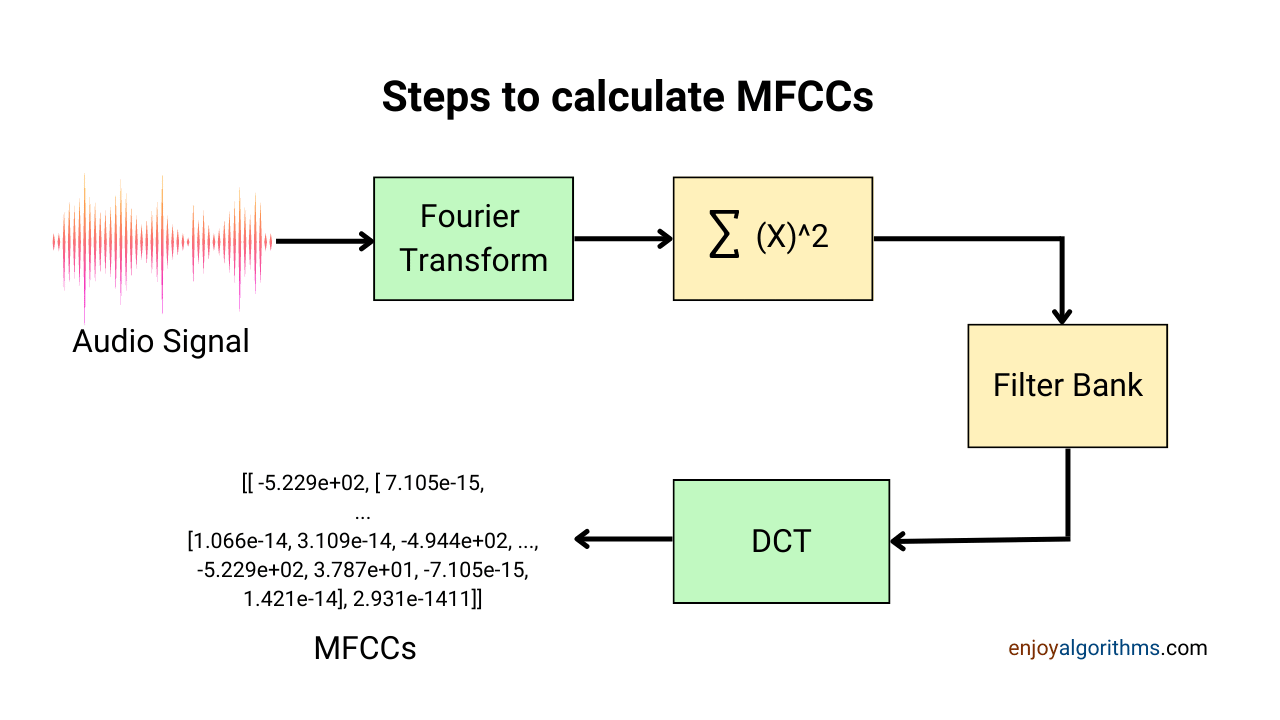We have explored both techniques and reached the stage where model development can take place. So let’s jump to the next section.

### Model development

Our major work has been done till now, i.e. spectrogram formation and MFCCs extraction. Now we will follow the standard steps of Neural network formation: Image preprocessing, Data splitting, Model formation, and Model Evaluation.

Our dataset has 600 audio clips of 3 seconds each of 6 different instruments:

``````class_names = [‘flute’, ‘viola’, ‘cello’, ‘oboe’, ‘trumpet’, ‘saxophone’]

instruments = {‘flute’:1, ‘viola’:2, ‘cello’:3, ‘oboe’:4, ‘trumpet’:5, ‘saxophone’:6}``````

We will convert these 600 audio clips to spectrograms and then train a neural network model to predict the instrument associated with the test audio clip.

### Image Preprocessing

There are no standard steps for image processing like text or tabular data. We usually convert the original image into either RGB format or Grayscale format. Both have their advantages and disadvantages.

#### RGB V/S Grayscale images

A raw image is converted to either RGB or Grayscale. RGB, as the name suggests, is an image having three colours: Red, Green, and Blue. It converts image data into three channels having a size of 8 bits each, with each pixel having 256 shades resulting in 16777216 combinations of colours. We generally use RGB format if our system is able to do complex calculations and is computationally strong. Due to its minute detailing, it is used in object detection-based applications.

In contrast, Grayscale is only a one-channel image and presents an image using shades of grey colour only. The image size is only 8 bits, and hence the computation is faster. Grayscale images are preferred if the colour information is not required, and only the intensity representation will do the work.

We converted the spectrograms to grayscaled images to keep our model simple. The image size of the spectrogram is also significant, which if converted to RGB format, will be computationally heavy for the model. The shape of the spectrogram is (480,640), which will become (307200, 1) after flattening. Now imagine if we use RGB images, this number will increase by three times because of the three channels.

``````instruments = {'flute':1, 'viola':2, 'cello':3, 'oboe':4, 'trumpet':5, 'saxophone':6}

for file in files:
name=name.split('\\')[-1]
grayimage = rgb2gray(img[...,0:3])
pixels = np.array(grayimage).flatten()
data.append(pixels)

labels.append(instruments[name] - 1)

data, labels = np.array(data), np.array(labels)
labels=labels.reshape(600,1)
labels2 = np.zeros((labels.shape, 6))

for i in range(labels.shape):

labels2[i][labels[i]] = 1``````

In this section, we converted the images to Grayscale and flattened them to a 1D array. Simultaneously, we appended these image arrays to the data array and the labels to the label array. After processing all the images, we encoded the labels using a one-hot encoding scheme, which we hard-coded in this case. Now we reached the stage where we can split our dataset into train and test data.

### Model formation

These steps are common for the data we prepared using the spectrogram approach and the MFCC approach. We will focus on the Artificial Neural Network Model formation in this section.

#### Deciding the number of layers and neurons

There is a saying, “Why use the sword if you can cut an apple with a knife.”. The same fits here. Why make a complex model if a simple model can work? We used two hidden layers with 64 and 32 neurons, respectively. There are six instrument classes, so the final layer will contain six neurons.

#### Deciding the activation function

We use a softmax or sigmoid activation function for classification problems in the output layer. For the hidden layers, Relu and Selu are two good choices to start with. This will remain the same for both approaches. To explore more about activation functions, you can refer to this blog.

#### Selecting the loss function and optimization algorithm

For multi-class classification, categorical-cross-entropy is best suited and commonly used loss function in Artificial Neural Networks.

For choosing the optimization algorithm, we should ask about the dataset size, the system’s computational power and whether we want to tune the learning rate manually. After this, we will determine which optimization algorithm is perfect for our model. I used Adam (Adaptive Moment Estimation) algorithm because it is swift and converges quickly.

#### Deciding the Epoch value

This part is interesting for this model. We generally use 200–1000 epoch values to train the model. But here, if we try to train this model for large epoch values, we might utilize all the system's cores and space. This is because of flattening the spectrograms to (307200, 1) size. Now you will agree that using Grayscaled images was a good idea instead of RGB images.

We used 15–20 epoch values for the spectrogram approach and 500–700 epoch values for the MFCC approach.

Now let’s quickly summarise these steps in the code.

``````X_train, X_test, y_train, y_test = train_test_split(data, labels2, test_size = 0.2, random_state = 0)

from keras.layers import Dense,Dropout,Activation,Flatten
model = tf.keras.models.Sequential()

from keras.layers import Dense, Activation
model = tf.keras.models.Sequential()

###second layer
###final layer

model.fit(X_train,y_train,epochs=5,)
loss,accuracy=model.evaluate(X_test,y_test,verbose=0)
y_pred=model.predict(X_test)

print('Accuracy: %.2f' % (accuracy*100))``````

### Model Evaluation

Evaluation metric measures how well the model performs on train and test data. For classification problems, the confusion matrix, accuracy, F1 score, and area under the curve (AUC) are the most common metrics. To get a detailed overview of evaluation metrics, hit this link.

We will evaluate and compare both approaches in this section. Let’s see the spectrogram approach first.

``````# Spectrogram Approach
loss,accuracy=model.evaluate(X_test,y_test,verbose=0)
y_pred=model.predict(X_test)

print('Accuracy: %.2f' % (accuracy*100))``````

Accuracy: 99.50

We perfectly achieved near to 100% accuracy with our model, but to be sure about the results, we have one more metric to evaluate the model performance.

#### Confusion Matrix

The confusion matrix divides predictions into four classes true positive, true negative, false positive, and false negative and helps us to understand the biases of the model. We have six classes depicted by a 6X6 matrix, as shown below.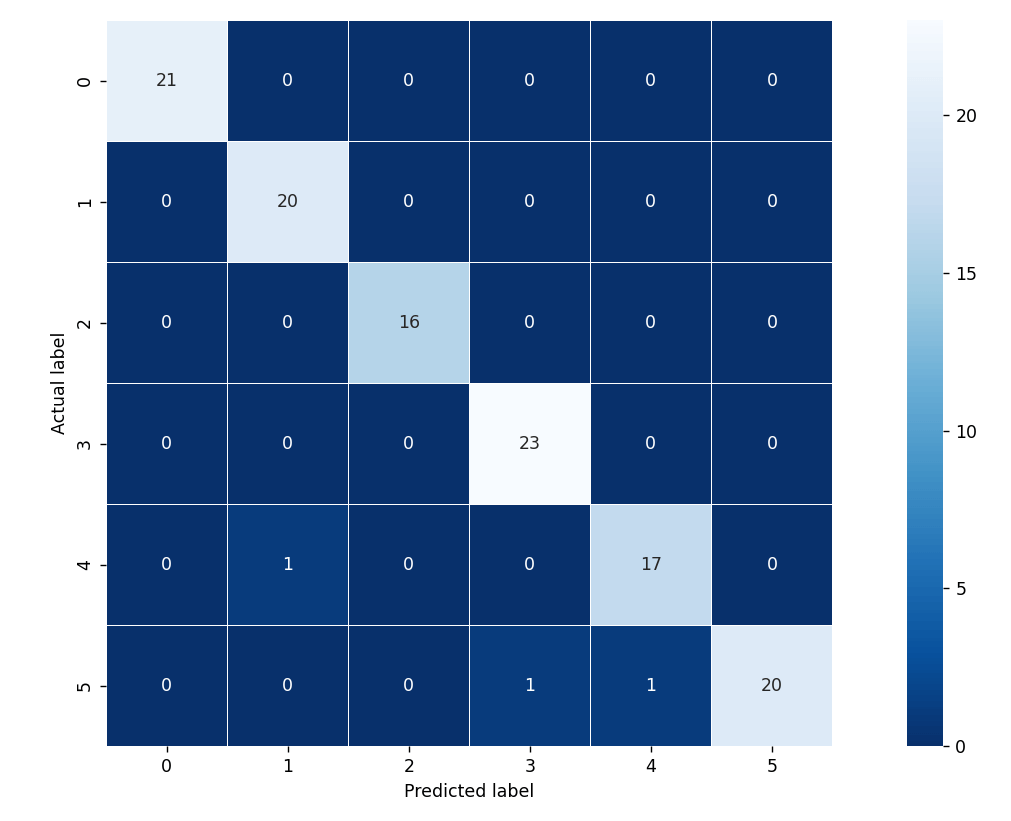Confusion Matrix for Spectrogram approach

We can see that only three samples got misclassified in the test data. This validates the accuracy score. Let's see the MFCC approach scores now. We have trained this model with three hidden layers instead of two and 500 epochs.

``````model.add(tf.keras.layers.Dense(units=64, activation='relu',))

model.fit(X_train,y_train,epochs=500)

loss,accuracy=model.evaluate(X_test,y_test,verbose=0)
y_pred=model.predict(X_test)

print('Accuracy: %.2f' % (accuracy*100))``````

Accuracy: 99.17

This model is also achieving an accuracy of 99%. This proves that both techniques are efficient and reliable.

### Applications of audio classification

Music information retrieval(MIR) is a vast field and most popular at the present time. It's a good time to put your hands on this data type and do something extraordinary with it. Here are some applications to help you master audio preprocessing.

• Genre Classification
• Mood Classification
• Artist Identification
• Instrument Recognition
• Music Annotation

We have explored only one application in this blog. There are four other applications you can explore using the same approach used in this blog. Feel free to reach us for any help!

### Conclusion

In this blog, we tried to open the gates of audio processing techniques for our learners. We discussed the two most famous techniques: Spectrogram and MFCs, and understood the relation between them and the procedure to generate them.

We also understood the difference between RGB and Grayscaled images. At last, we followed the standard steps to make an Artificial Neural Network model for the music instrument recognition task and achieved 99% accuracy.

##### Enjoy Learning!

☆ 16-Week Live DSA Course
☆ 10-Week Live DSA Course